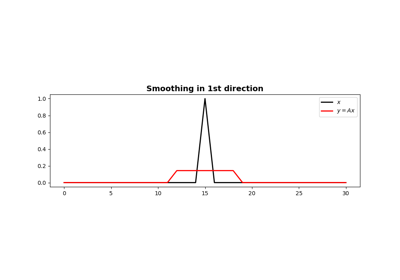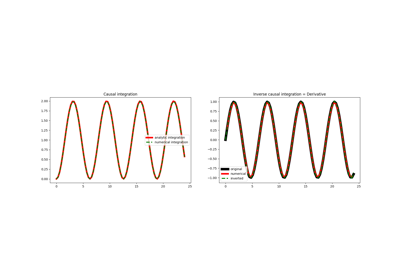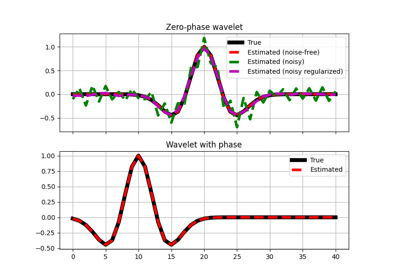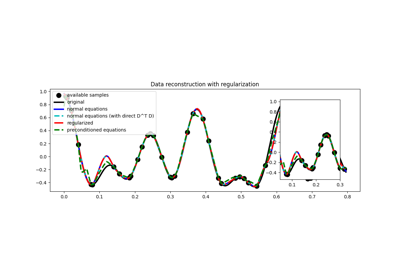# pylops.Smoothing1D¶

pylops.Smoothing1D(nsmooth, dims, dir=0, dtype='float64')[source]

1D Smoothing.

Apply smoothing to model (and data) along a specific direction of a multi-dimensional array depending on the choice of dir.

Parameters: nsmooth : int Lenght of smoothing operator (must be odd) dims : Number of samples for each dimension dir : int, optional Direction along which smoothing is applied dtype : str, optional Type of elements in input array.

Notes

The Smoothing1D operator is a special type of convolutional operator that convolves the input model (or data) with a constant filter of size $$n_\text{smooth}$$:

$\mathbf{f} = [ 1/n_\text{smooth}, 1/n_\text{smooth}, ..., 1/n_\text{smooth} ]$

When applied to the first direction:

$y[i,j,k] = 1/n_\text{smooth} \sum_{l=-(n_\text{smooth}-1)/2}^{(n_\text{smooth}-1)/2} x[l,j,k]$

Similarly when applied to the second direction:

$y[i,j,k] = 1/n_\text{smooth} \sum_{l=-(n_\text{smooth}-1)/2}^{(n_\text{smooth}-1)/2} x[i,l,k]$

and the third direction:

$y[i,j,k] = 1/n_\text{smooth} \sum_{l=-(n_\text{smooth}-1)/2}^{(n_\text{smooth}-1)/2} x[i,j,l]$

Note that since the filter is symmetrical, the Smoothing1D operator is self-adjoint.

Attributes: shape : tuple Operator shape explicit : bool Operator contains a matrix that can be solved explicitly (True) or not (False)

## Examples using pylops.Smoothing1D¶1D SmoothingCausal IntegrationWavelet estimation03. Solvers